# Op Amp Design For Pressure Transducer

#### Avenues

Joined Nov 30, 2015
7
Hello,

I was hoping I could get some help with a project I'm working on. I have a pressure transducer that I want to run into my A/D converter to get chamber pressure on a rocket motor I'm building, however to get the best resolution I want to build an amplifier. I'll state up front I know very little about designing circuits but I've been trying to do my homework....

With that said, I want to build an op amp with a gain of 4 and offset of -9v. My A/D converter is the DI-145 which accepts +/-10V with 10 bit resolution, my 1600psi transducer runs on 5v and outputs .v = 0psi and to 4.5v = 1600psi. I think I could get good data without an amp, however if I can build one that has a gain of 4 with a -9v offset I would have 4x the resolution. Attached is my first stab at the schematic, any advice would be greatly appreciated! Thanks!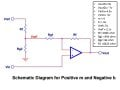#### MikeML

Joined Oct 2, 2009
5,444
Something wrong with your math. If the input range is -7V to +9V, and you want -10V to +10V out, then the gain must be (10-(-10))/(9-(-7)) = 20/16 = 1.25. Since you are using a non-inverting stage, the gain is (1+R2/R1), so if R1 is 100K, R2=25K.

The mean value of the input range is (-7+9)/2 = 2/2 = 1. The mean value is amplified by 1.25, so causes a 1.25V offset in the output.
Since the gain from the left end of R1 is -R2/R1 = -25K/100K = -0.25, then if you apply 5V there, that will cause a -1.25V shift of the output.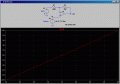Obviously, you must use an opamp with +-15V split supplies.

#### Attachments

• 1 KB Views: 4
Last edited:

#### Avenues

Joined Nov 30, 2015
7
Thanks for the response Mike, however let me try to clear up the values. -7v to +9v is the desired amp output, not input. The low input is 0.5v while the high end input is 4.5v. My desired output for these values is -7v for the low end and +9v for the high end:

(0.5*4) – 9 = -7

and

(4.5*4) – 9 = 9

where gain = 4 and offset = -9

I think the math is correct however there may be a flaw in my approach. I did see in my original paragraph that there is one typo where the low end input reads 0 psi = .v where is should 0 psi = .5v however I cannot edit it for some reason. Thanks for your help.

#### MikeML

Joined Oct 2, 2009
5,444
If the input range is 0.5V to 4.5V, and you want -7V to +9V out, then the gain must be (9-(-7))/(4.5-0.5) = 16/4 = 4. Since you are using a non-inverting stage, the gain is (1+R2/R1), so if R1 is 50K, R2=150K.

The mean value of the input range is (0.5+4.5)/2 = 5/2 = 2.5. The mean value is amplified by 4, so causes a 10V offset in the output.

You want the output range centered at the mean value of the output range. (9+(-7))/2 = 1V, so you want a net -9V shift at the output.

Since the gain from the left end of R1 is -R2/R1 = -150K/50K = -3, then if you apply -9/-3V = 3V, that will cause a -9V shift of the output.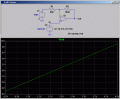However, you do not have a 3.000V reference; you have 5.000V. One option is use a voltage divider between 5.000V and Gnd, and then buffer the tap with an opamp voltage follower.There exist two resistors R1 and R2 that would do what you need that looks like this: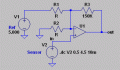I will let you come up with the actual values. You get to assume one of the values, for example R3=150K, and the other two depend on R3. An exercise in solving two simultaneous equations in two unknowns.

Last edited:

#### wayneh

Joined Sep 9, 2010
17,201
I'm guessing you won't have an easy way to calibrate this at 1600psi, so I'd be inclined to use an accurate and stable voltage reference instead of relying on a resistive voltage divider.

#### MikeML

Joined Oct 2, 2009
5,444
... so I'd be inclined to use an accurate and stable voltage reference instead of relying on a resistive voltage divider.
@wayneh ,

TS is using a 5.00V reference voltage source. Both circuits I posted are perfectly ratiometric with respect to the 5.00V reference. How would you improve on it?

@Avenues ,
Here is one combination that works: (Can you say Thevenin Equivalent?)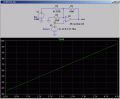Last edited:

#### wayneh

Joined Sep 9, 2010
17,201
How would you improve on it?
To my way of thinking, when there is a resistor between the reference and the op-amp, you no longer have a reference voltage at the amp. I know you can buy good resistors with high precision and low temperature coefficients, and you could include a trim pot for adjustment, but isn't there a way to connect the op-amp directly to the reference?

#### MikeML

Joined Oct 2, 2009
5,444
To my way of thinking, when there is a resistor between the reference and the op-amp, you no longer have a reference voltage at the amp. I know you can buy good resistors with high precision and low temperature coefficients, and you could include a trim pot for adjustment, but isn't there a way to connect the op-amp directly to the reference?
As long as the tempco of the resistors is matched, then the output from a voltage divider made from such resistors preserves the precision of the original reference.

#### wayneh

Joined Sep 9, 2010
17,201
I guess the thing that confused me was how the voltage applied to the inverting input is not always Vref but depends on the amp's output voltage. I was thinking that was a bad thing but I get it now.

#### Avenues

Joined Nov 30, 2015
7
Thanks for the discussion guys. Can you recommend a specific amp? I was considering a TL084 however i I'm open to suggestions.

#### wayneh

Joined Sep 9, 2010
17,201
The last op amp I bought was a TLV272. It's rail-to-rail, so maybe worth a look here.

#### Avenues

Joined Nov 30, 2015
7
Thanks everyone for the help. Hope to get my stuff soon and start right away.

#### Avenues

Joined Nov 30, 2015
7
So I tried sourcing the parts at my local electronics store and had to make some changes to what I originally intended building. With what they had available I ended up using Mike's earlier layout with the 3v reference instead of the 5v, I simply tweaked my voltage regulator to supply 3.00v instead of 5.00v. As far as op amps all I could find was a LM741 which appeared to fit the bill.

The first image is Mike's schematic that I intended to build, while the second image is my attempted implementation using the LM741, does this look ok?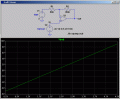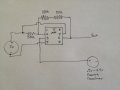For reference.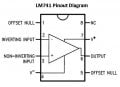Last edited by a moderator:

#### MikeML

Joined Oct 2, 2009
5,444
The circuit I posted uses an "idealized" opamp where neither the power supply connections, nor trimming, nor bypass capacitors are shown.

To get -7V output with a crummy old '741, your negative supply must be at least or more negative than -10V.

To get +8V output with a crummy old '741, your positive supply must be at least or more positive than +11V.

Normally, the '741 is operated on +-15V split supplies...

#### Avenues

Joined Nov 30, 2015
7
Duh, ok thanks for clarification. I have it working on the breadboard however I need to add the low pass filtering as the output signal is a bit noisy.

I believe the C = 1/(2*Pi*R2*Fc) and since I'm sampling at no more than 240Hz and R2= 150K ohms I'm getting 4420 pF for the capacitor running over R2. Am I'm heading in the right direction? If it makes part selection easier I could lower the sampling rate by half and probably be fine.